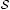﻿ ﻿Notation - Appendices - Computer Vision: Models, Learning, and Inference (2012)

# Computer Vision: Models, Learning, and Inference (2012)

### Notation

This is a brief guide to the notational conventions used in this text.

Scalars, vectors, and matrices

We denote scalars by either small or capital letters a, A, α. We denote column vectors by bold small letters a,. When we need a row vector we usually present this as the transpose of a column vector aT,T.

We represent matrices by bold capital letters B, Φ. The ith row and jth column of matrix A is written as aij. The jth column of matrix A is written as aj. When we need to refer to the ith row of a matrix, we write this as ai• where the bullet • indicates that we are considering all possible values of the column index.

We concatenate two D × 1 column vectors horizontally as a = [b, c] to form the D × 2 matrix A. We concatenate two D × 1 column vectors vertically as a = [bT, cT]T to form the 2D × 1 vector a. Although this notation is cumbersome, it allows us to represent vertical concatenations within a single line of text.

Variables and parameters

We denote variables with Roman letters a, b. The most common examples are the observed data which is always denoted by x and the state of the world which is always denoted by w. However, other hidden or latent variables are also represented by Roman letters. We denote parameters of the model by Greek letters μ, Φ, σ2. These are distinguished from variables in that there is usually a single set of parameters that explains the relation between many sets of variables.

Functions

We write functions as a name, followed by square brackets that contain the arguments of the function. For example, log[x] returns the logarithm of the scalar variable x. Sometimes we will write a function with bullets • as arguments (e.g., atan2[•,•]) to focus the interest on the function itself rather than the arguments.

When the function returns one or more vector or matrix arguments, it is written in bold. For example, the function aff[x, Φ, τ] applies an affine transformation to the 2D point x with parameters Φ, τ and returns a new 2D vector output. When a function returns multiple outputs, we write this in Matlab notation so [U,L,V] = svd[X] returns the three parts U,L,V of the singular value decomposition of X.

Some functions are used repeatedly throughout the text. These include:

• minx f[x], which returns the minimum possible value of the function f[x] as we vary x over its entire valid range,

• argminx f[x], which returns the value of the argument x that minimizes f[x],

• maxx and argmaxx, which fulfill the same roles as minx and argminx but where we are maximizing the function,

diag[A], which returns a column vector containing the elements on the diagonal of matrix A,

δ[x] for continuous x, which is a Dirac delta function and has the key property f[x] δ[xx0]dx = f[x0],

δ[x] for discrete x, which returns 1 when the argument x is 0 and returns 0 otherwise, and

• heaviside[x], which represents the Heaviside step function. It returns 0 when the argument x < 0 and returns 1 otherwise.

Probability distributions

We write the probability of a random variable x as Pr(x). We write the joint probability of two variables a,b as Pr(a, b) and the conditional probability of a given b as Pr(a|b). Sometimes, we wish to specify the exact value b* that a is conditioned upon and here we write Pr(a|b = b*). Occasionally, we denote that variables a and b are independent by writing a ⊥⊥ b. Similarly we indicate that a and b are conditionally independent given c by writing a ⊥⊥ b|c.

Probability distributions are written in the style Pr(x|μ,) = Normx[μ,]. This returns the value of the multivariate normal distribution for data x when the distribution has mean μ and covariance . In this way, we always distinguish the argument of the distribution (here x) from the parameters (here μ,).

Sets

We denote sets with calligraphic letters. The notationindicates thatis a subset of. The notation xindicates that x is a member of the set. The notation=indicates that setis the union of setsand. The notation=\indicates that setconsists of all of the elements ofexcept those that are in.

Often we write out a set explicitly in terms of the elements and for this we use curly brackets so that= {x,y,z} indicates that the setcontains x,y, and z and nothing else. When a set is empty, we write= {}. We use the notation {xi}Ii=1 as shorthand to represent the set {x1,x2,…,xI}, and we may write the same set in the compact form x1…I if it is part of an equation.

﻿

All materials on the site are licensed Creative Commons Attribution-Sharealike 3.0 Unported CC BY-SA 3.0 & GNU Free Documentation License (GFDL)